# Subgroup control chart in Excel tutorial

2017-10-20

This tutorial will help you set up and interpret a subgroup control chart on quantitative data in Excel using the XLSTAT statistical software.

## Dataset to generate a subgroup chart

An Excel sheet containing both the data and the results for use in this tutorial can be downloaded by clicking here.

The data are from [Pyzdek Th. (2003); The Six Sigma Handbook, McGraw Hill, New York] and correspond to 25 inspections each having 5 measurements of a production process.

## Subgroup charts

The control charts are an efficient method to decide, if a process is statistically under control or not. There is a variety of different control charts that can be used for different aims.

The subgroup charts tool offers you the following chart types alone or in combination:

• X bar
• R
• S
• S’²

An X bar chart is useful to follow the mean of a production process. Mean shifts are easily visible in the diagrams.

An R chart (Range diagram) is useful to analyze the variability of the production. Large differences in production, caused by the use of different production lines, will be easily visible.

S and S’² charts are also used to analyze the variability of production. The S chart draws the standard deviation of the process and the S’² chart draws the variance (which is the square of the standard deviation).

Note 1: If you want to investigate smaller mean shifts, then you can also use CUSUM group charts which are often preferred in comparison to the subgroup control charts, because they can detect smaller mean shifts.

Note 2: If you have only one measurement for each point in time, then please use the control charts for individuals.

Note 3: If you have measurements as qualitative values (for instance ok, not ok, conform not conform), then use the control charts for attributes.

In addition, you will find in XLSTAT functions that are useful to improve the analysis:

• Box-Cox transformation
• Process capability
• Tests for normality
• Rules for special causes and Westgard rules
• Run charts

During this tutorial an X-bar chart together with an R chart are generated.

## Setting up subgroup charts

Once XLSTAT is activated, select the XLSTAT / SPC / Subgroup charts command.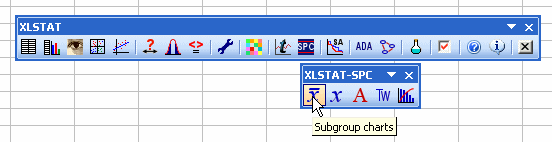The Statistical Process Control / Subgroup dialog box appears

In the Mode tab, we choose the combination X-bar — R chart.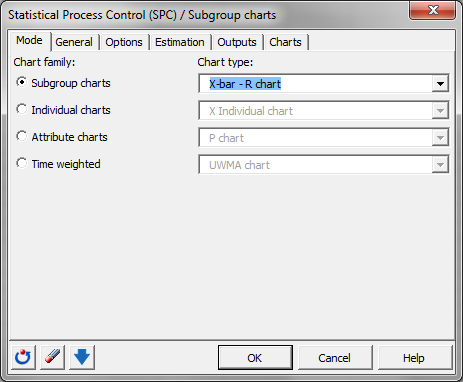Then move on to the tab General and select the data on the Excel sheet. (Note: There are several ways for selecting data with XLSTAT - for further information, please check the section on selecting data.)

In this example, the data start from the first row, so it is quicker and easier to use columns selection. This explains why the letters corresponding to the columns are displayed in the selection boxes. The Data format chosen is Columns because of the format of the input data.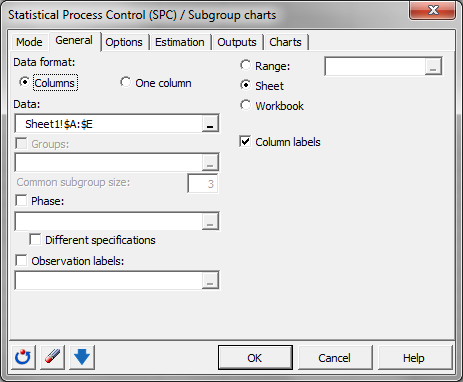In the Options tab, we activate the options Process capabilities to display the process key figures. The given values for the USL, LSL and the target are entered.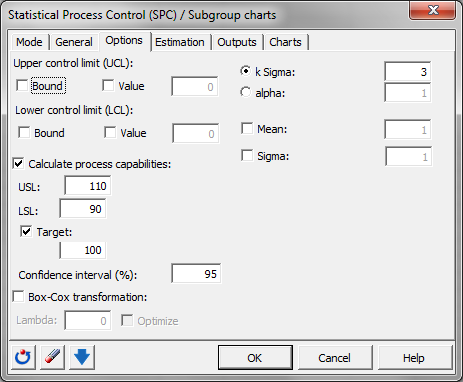In the Estimation tab, we chose the option R-bar.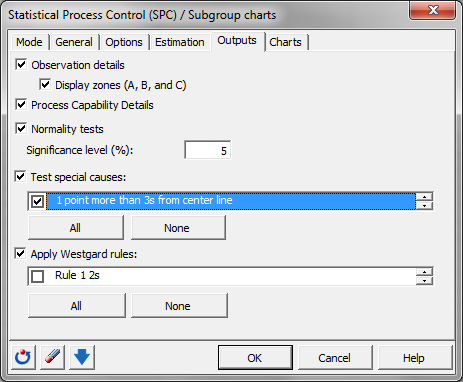In the Output tab, we activate all options, and we activate all special cause rules.In the Charts tab, we activate all options.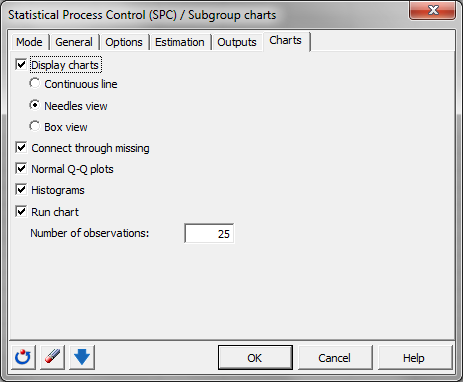The computations begin once you have clicked on OK. You are asked to confirm the number of rows and columns (this message can be bypassed by unselecting the Ask for selections confirmation in the XLSTAT Options tab).

## Interpreting the subgroup charts

The first results that are displayed are the estimated mean and the standard deviation. Then, the process capability figures together with a chart and the X-bar control limits are displayed. It is interesting to see that the Cp indicator tells the process is "not adequate". We see the specification limits (red) are wider than the control limits of the X-bar chart (blue). The process is not capable according to the specifications.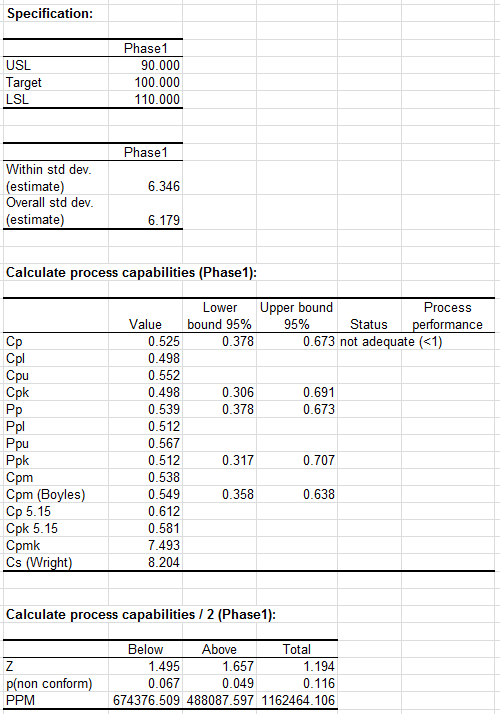The following tables and the corresponding chart represent the X-bar control chart including the different control limits and central lines.

In the first table the data of the X-bar control chart starting with the group mean, group min and group max are displayed. Then, the central line (CL), the lower (LCL) and upper (UCL) control limits, and the lower and upper zone limits for the A and B areas are displayed for each group.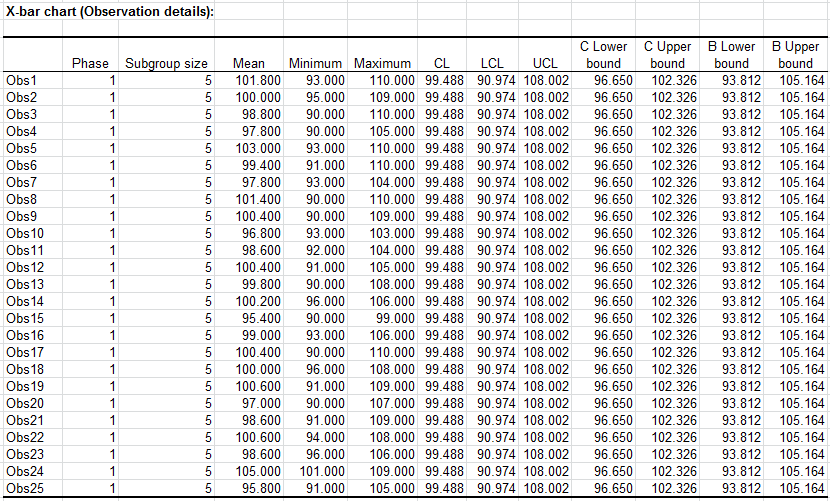The next table contains the details for the special cause rules. Only "No" can be read in the table. The data has a good quality with respect to this issue. Summing up the conclusions made above, you can discover the different things suggested by the X bar control chart. The group means values are always between the lower and upper control limits.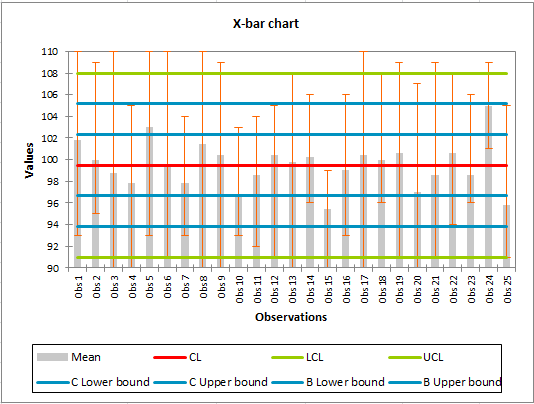Similar to the X-bar chart, the data of the R chart are always inside the control limits and no special causes are present.

Both control charts let us come to the conclusion that the process is “statistically under control”.

Further it is interesting to know, if the data follows a normal distribution and therefore the common rules for control charts and process capabilities can be applied. In the next section the results of 4 different normality tests and a Q-Q plot are displayed. All of the 4 tests conclude that the Hypothesis H0 of normal distribution can be accepted. In the Q-Q plot we see that the data are close to the first bisector line. We suppose that the data are normally distributed.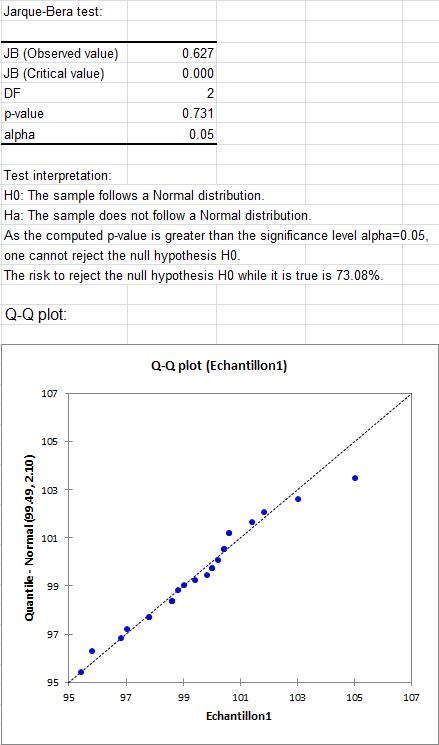Finally, the run chart is displayed. It contains all individual measurements in order to judge the range and trends. We see that the measurements inside a group have a wide range, lying between 91 and 110. Therefore the group means representing these measurements has to be used carefully. This is the reason why the Cp value of the process indicates a process that has not a good performance. This is caused by the values of the specifications.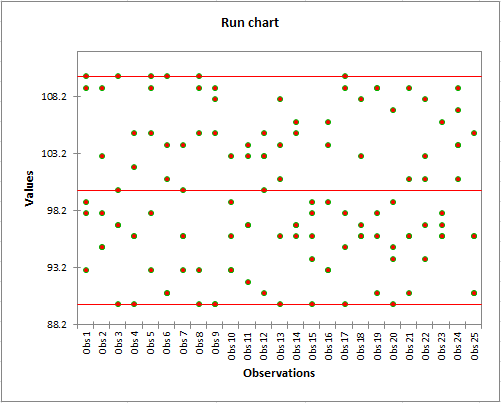#### Contact our technical support team: support@xlstat.com

https://cdn.desk.com/
false
desk